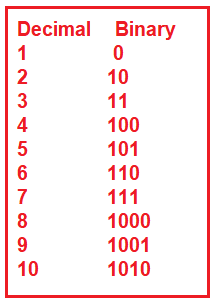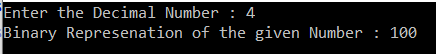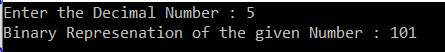# Decimal to Binary Conversion in C#

## Decimal to Binary Conversion in C# with Examples

In this article, I am going to discuss the Decimal to Binary Conversion in C# with some examples. Please read our previous article where we discussed the Sum of Digits of a given number Program in C# with many different ways. In C#, we can easily convert any decimal number (base-10 (i.e. 0 to 9)) into binary number (base-2 (i.e. 0 or 1)). As part of this article, we are going to discuss the following pointers.

1. What are Decimal Numbers?
2. What are Binary Numbers?
3. How to Convert Decimal to Binary in C#?
##### What are Decimal Numbers?

The Decimal numbers are the numbers whose base is 10. That means the decimal numbers are ranges from 0 to 9. Any combination of such digits (digits from 0 to 9) is a decimal number such as 2238, 1585, 227, 0, 71, etc.

##### What are Binary Numbers?

The Binary numbers are the numbers whose base is 2. That means the binary numbers can be represent using only two digits i.e. 0 and 1. So, any combination of these two numbers (i.e. 0 and 1) is a binary number such as 101, 10o1, 111111, 10101, etc.

Let us have a look which shows the decimal number along with its binary representation.##### Algorithm: Decimal to Binary Conversion

Step1: First, divide the number by 2 through modulus (%) operator and store the remainder in an array
Step2: Divide the number by 2 through division (/) operator.
Step3: Repeat step 2 until the number is greater than zero.

##### Program: Decimal to Binary Conversion in C#
```using System;
namespace LogicalPrograms
{
public class Program
{
static void Main(string[] args)
{
Console.Write("Enter the Decimal Number : ");
int i;
int[] numberArray = new int;
for (i = 0; number > 0; i++)
{
numberArray[i] = number % 2;
number = number / 2;
}

Console.Write("Binary Represenation of the given Number : ");
for (i = i - 1; i >= 0; i--)
{
Console.Write(numberArray[i]);
}

}
}
}
```

Output:##### Let us see another way of doing the conversion from Decimal to Binary in C#.

In the following program, we created a string variable to hold the binary representation of the Decimal number.

```using System;
namespace LogicalPrograms
{
public class Program
{
static void Main(string[] args)
{
Console.Write("Enter the Decimal Number : ");

string Result = string.Empty;
for (int i = 0; number > 0; i++)
{
Result = number % 2 + Result;
number = number / 2;
}
Console.WriteLine(\$"Binary Represenation of the given Number : {Result}");